# Ex. 1

## (a) Using “scan”

• Use scan() to read every single cell in the baseball-salaries-2016.txt file, need to set sep="\t" to avoid a 2-gram term from being separated into two words.
• Use data.frame() to create a data.frame (class) by the input vector.
• Then use write.csv() and write.table() to export the file.

• salary = Salary ($M) • winning percentage (WPCT) = W/(W+L)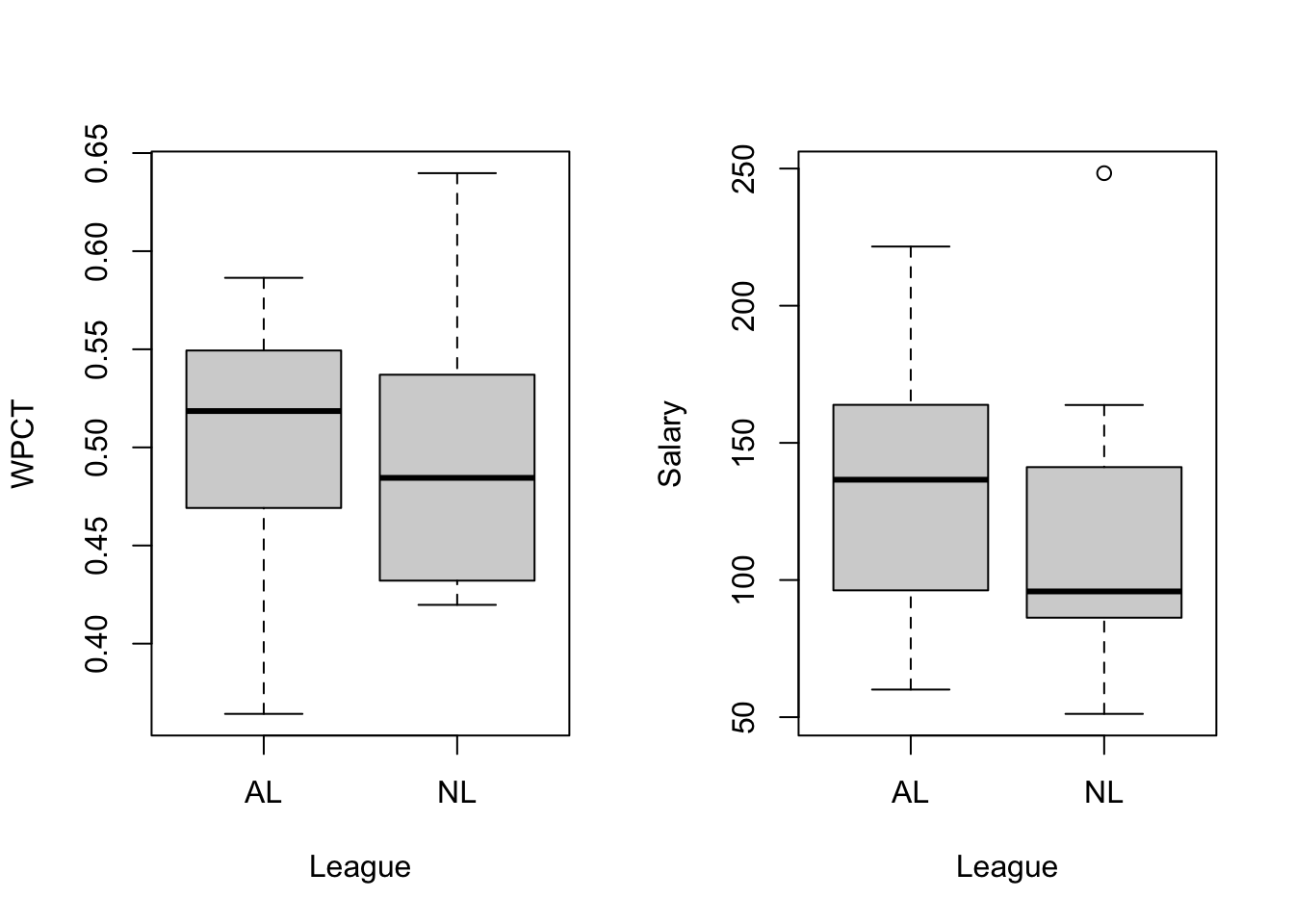## (c) Check correlation • Using cor.test() to check whether the positive relations between salary and winning percentage. • The Pearson’s correlation between salary and winning percentage is 0.617, p<.001, so we can reject the null hypothesis, i.e. the correlation between WPCT and salary is significant. ## ## Pearson's product-moment correlation ## ## data: sala and wpct ## t = 4.1436, df = 28, p-value = 0.0002856 ## alternative hypothesis: true correlation is not equal to 0 ## 95 percent confidence interval: ## 0.3294329 0.7992681 ## sample estimates: ## cor ## 0.6165296 ## (d) Scatter plot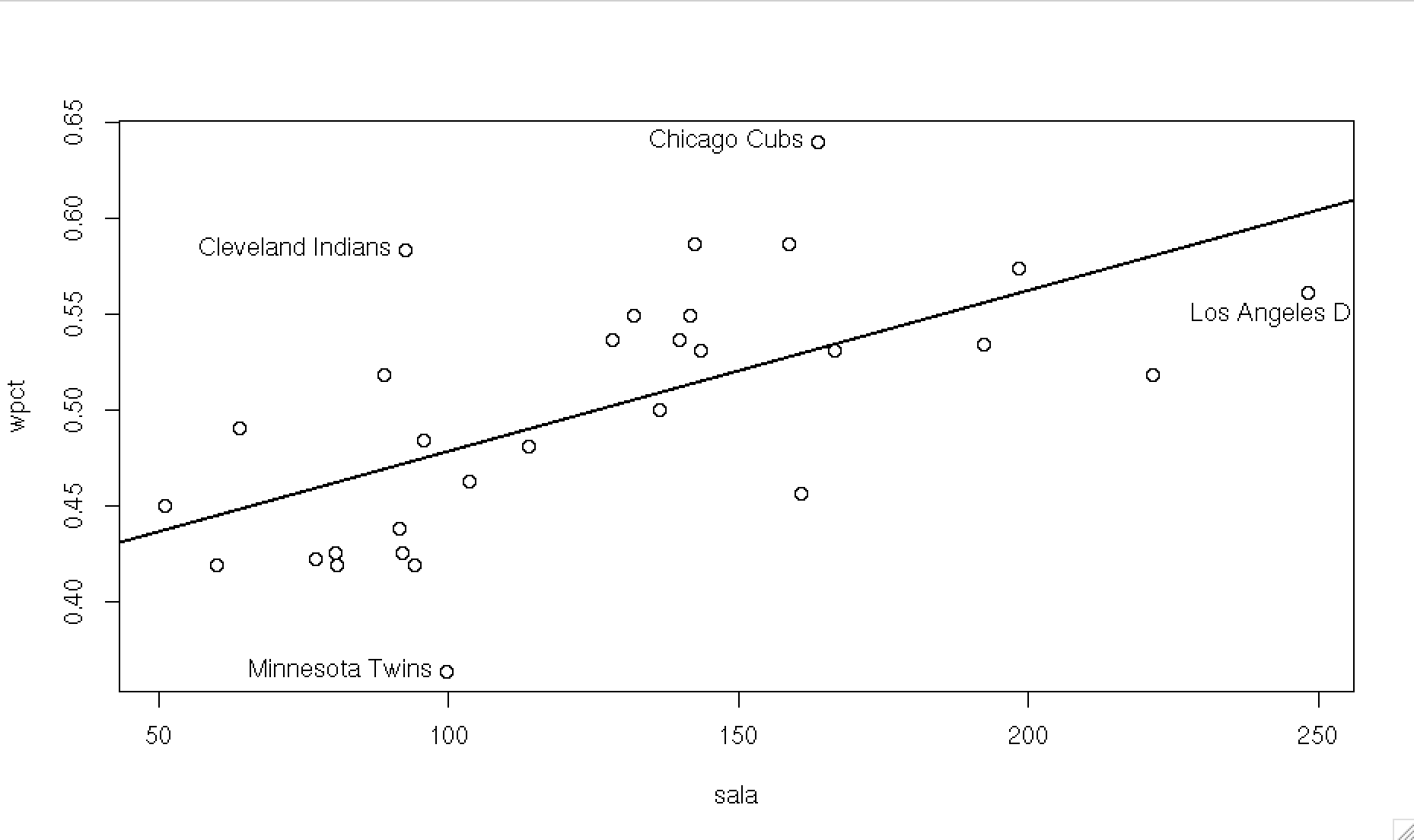# Ex. 2 ## (a) Length of a vector The sample is shown below. When we shorten a vector (set a random seed) from length 20 to 15, only the first 15 numbers would be present. When we lengthen it to 18, three (18-15=3) NAs would be added. • the results of rnorm(20). ##  -1.21 0.28 1.08 -2.35 0.43 0.51 -0.57 -0.55 -0.56 -0.89 ##  -0.48 -1.00 -0.78 0.06 0.96 -0.11 -0.51 -0.91 -0.84 2.42 • the results of length(x) = 15 ##  -1.21 0.28 1.08 -2.35 0.43 0.51 -0.57 -0.55 -0.56 -0.89 ##  -0.48 -1.00 -0.78 0.06 0.96 • the results of length(x) = 18 ##  -1.21 0.28 1.08 -2.35 0.43 0.51 -0.57 -0.55 -0.56 -0.89 ##  -0.48 -1.00 -0.78 0.06 0.96 NA NA NA ## (b) Operations of a vector • Change the $$x<0$$ part of x[1:20] to NA. The results is shown below. ##  NA 0.28 1.08 NA 0.43 0.51 NA NA NA NA ##  NA NA NA 0.06 0.96 NA NA NA NA 2.42 • computing the the mean, median, and variance of non-NA entries in x[1:18] from (a). ##  "mean: 0.55" ##  "median: 0.47" ##  "var: 0.16" ## (c) Change NA to 0 • using is.na() to identify the NAs, then replaced them by 0s. ##  0.00 0.28 1.08 0.00 0.43 0.51 0.00 0.00 0.00 0.00 ##  0.00 0.00 0.00 0.06 0.96 0.00 0.00 0.00 0.00 2.42 # Ex. 3 ## (a) Graph The graph is shown as 3.(b) below. ## (b) Tangent line Find the slope and intercept of the tangent line of $$f(x)=x+2e^{(x-3)}+1$$ at $$x=5$$, then we can use abline() function to draw the tangent line. (Note. in the R abline() function, the intercept is denoted as a, and the slope is denoted as b.) • Slope. $$f'(5)=1+2e^{(5-3)}=1+2e^2$$ • Point. $$(x,y)=(5, f(5))=(5, 2e^2+6)$$ ( Note. $$x=5$$ substituted back into the original equation $$f(x)$$) • Intercept. based on $$y=bx+a$$, and $$b=(1+2e^2)$$ is known. (follow the parameters of abline().) $$2e^2+6=5(1+2e^2)+a$$, find $$a=-8e^2+1$$ .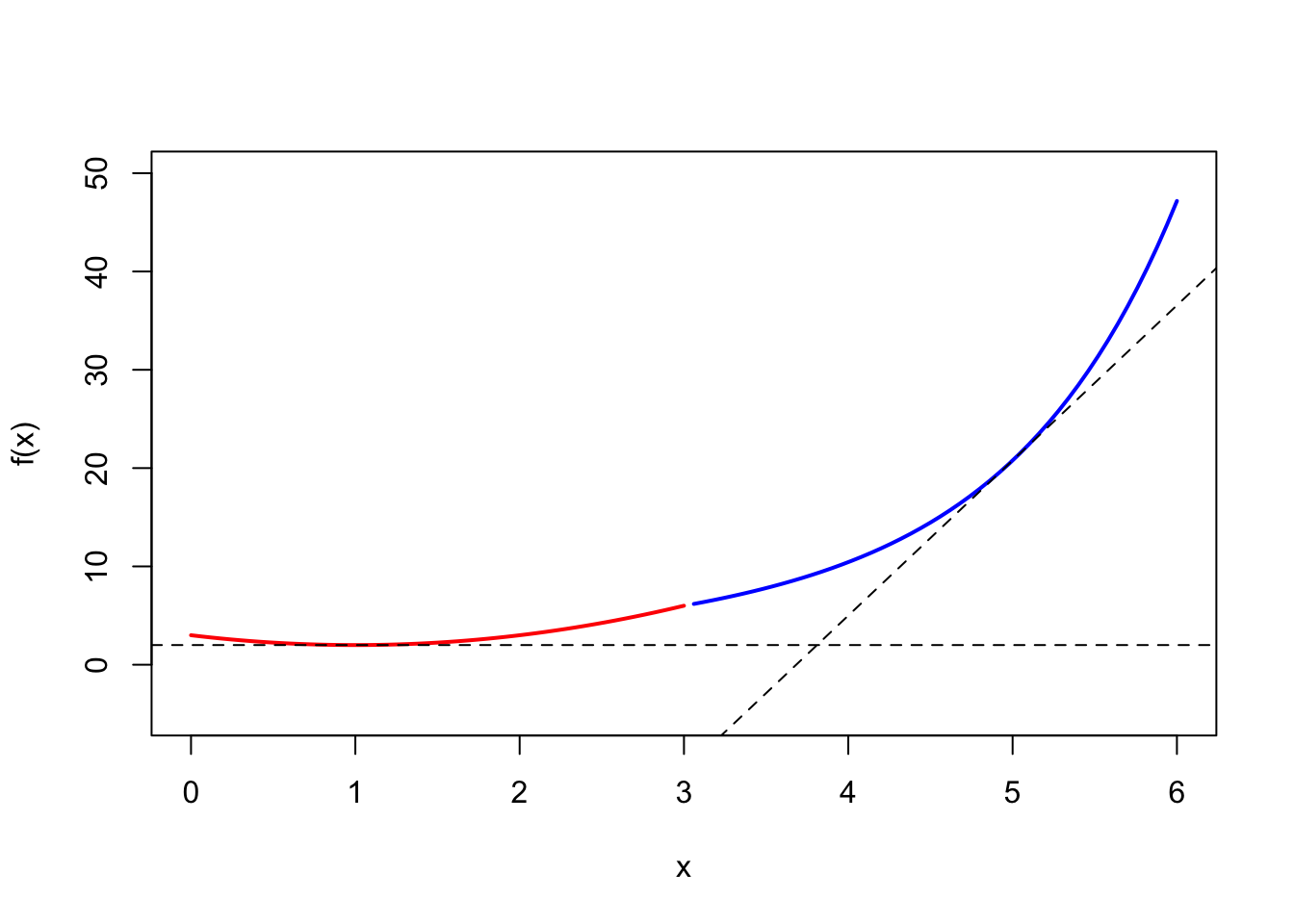# calculus expression((x^2-2*x+3), 'x') |> D('x') ## 2 * x - 2 expression((x+2*exp(x-3)+1), 'x') |> D('x') ## 1 + 2 * exp(x - 3) # Ex. 4 ## (a) cat() and date() The codes and outputs are shown below, combine the labels and system time by cat() function. cat( labels = "Today’s date is: ", paste( substr(date(),1,10), substr(date(),21,24)), fill = TRUE ) ## Today’s date is: Tue Mar 22 2022 cat( labels = "The time now is: ", paste( substr(date(),12,19)), fill = TRUE ) ## The time now is: 23:30:47 ## (b) Explain the outputs of time. • With the first 2 lines, we assign the system date to the object today (with a class “Date”), then display it as day-month-year format, and the month %b is an abbreviated month in English. • With the 3rd line, we create a sequence that has 10 days, begins today, and generates every other week. • In the 4th line, we extract the weekday name from the object today. In the 5th line, we check every single month’s name from the sequence tenweeks with 10 values. • In the last line, we check the leap seconds (潤秒) before, then display them as the date format. (today <- Sys.Date()) format(today, "%d %b %Y") (tenweeks <- seq(today, length.out=10, by="1 week")) weekdays(today) months(tenweeks) as.Date(.leap.seconds) # Ex. 5 ## (a) Mid-Square Method • By the requirements, the initial values is a 6 digits number, then square it. • How to make sure the 12-digits (square of 6-digits) at the middle of number (including 0, ranging over [0, 999999])? We have introduced the padding method from the stringr package. • The comment of the gen_5a() function. (1) Create a null vector vec. (2) In the for loop, we consider the input or iterate value may not be 6-digits, so we convert it to a character class first, then use str_pad() function to impute 0 at the left side until 12 digits. (3) Use str_sub() to extract the 6 digits from the 4th number to the 9th. (4) Convert the 6-digit number back into the numeric class. (5) Save the numbers in the vector vec with the ith place. • Goodness-of-fit test. We generated 10000 random numbers from the gen_5a() function. The K-S test showed D = 1, p-value < .001, so we reject the null hypothesis, i.e. the random numbers do not follow the uniform distribution. ## Warning in ks.test(res_5a, y = "punif"): ties should not be present for the ## Kolmogorov-Smirnov test ## ## One-sample Kolmogorov-Smirnov test ## ## data: res_5a ## D = 1, p-value < 2.2e-16 ## alternative hypothesis: two-sided ## (b) Vax’s generator • The comment of the gen_5b() function. (1) Create a null vector vec. (2) In the for loop, implement a Vax’s generator (69069*x) %% (2^32). (3) Save the numbers in the vector vec with the ith place. • Goodness-of-fit test. We generated 10000 random numbers from the gen_5b() function. For $$\chi^2$$ test, we separated the 10000 numbers to 10 groups by the intervals of 1/10 of range (max(res_5b) - min(res_5b))/k. Then check the K-S test D = 1, p < .001, we can reject the null hypothesis, i.e. the random numbers do not follow the uniform distribution. However, the $$\chi^2$$ test showed $$\chi^2=6.9936$$ with df=9, p=0.6378, we can not reject the null hypothesis, i.e. there are no significant difference between the random numbers and uniform distribution. • K-S test and $$\chi^2$$ test have different results. But K-S test considers each value, may be better than $$\chi^2$$ test. ## ## One-sample Kolmogorov-Smirnov test ## ## data: res_5b ## D = 1, p-value < 2.2e-16 ## alternative hypothesis: two-sided ## ## Chi-squared test for given probabilities ## ## data: chi_5b ## X-squared = 6.9936, df = 9, p-value = 0.6378 ## (c) Multiplicative congruential generators • The comment of the gen_5c() function. (1) Create a null vector vec. (2) In the for loop, implement a combined generators, (171*x) %% 30269, (172*y) %% 30307, (170*z) %% 30323, and (x/30269 + y/30307 + z/30323) %% 1. (3) Save the numbers in the vector vec with the ith place. • Goodness-of-fit test. We generated 10000 random numbers from the gen_5c() function. Check the K-S test D = 0.010053, p = 0.2643, we can not reject the null hypothesis, i.e. the random numbers do follow the uniform distribution. • Comparison of (a), (b) and (c). We can find the generator of 5(a) and 5(b) can not create a good random numbers iid from uniform distribution (based on K-S test). But the combination generator of 5(c) can do it, because it has a longer cycle. ## ## One-sample Kolmogorov-Smirnov test ## ## data: res_5c ## D = 0.010053, p-value = 0.2643 ## alternative hypothesis: two-sided The histograms of 5(a), 5(b) and 5(c) is shown below.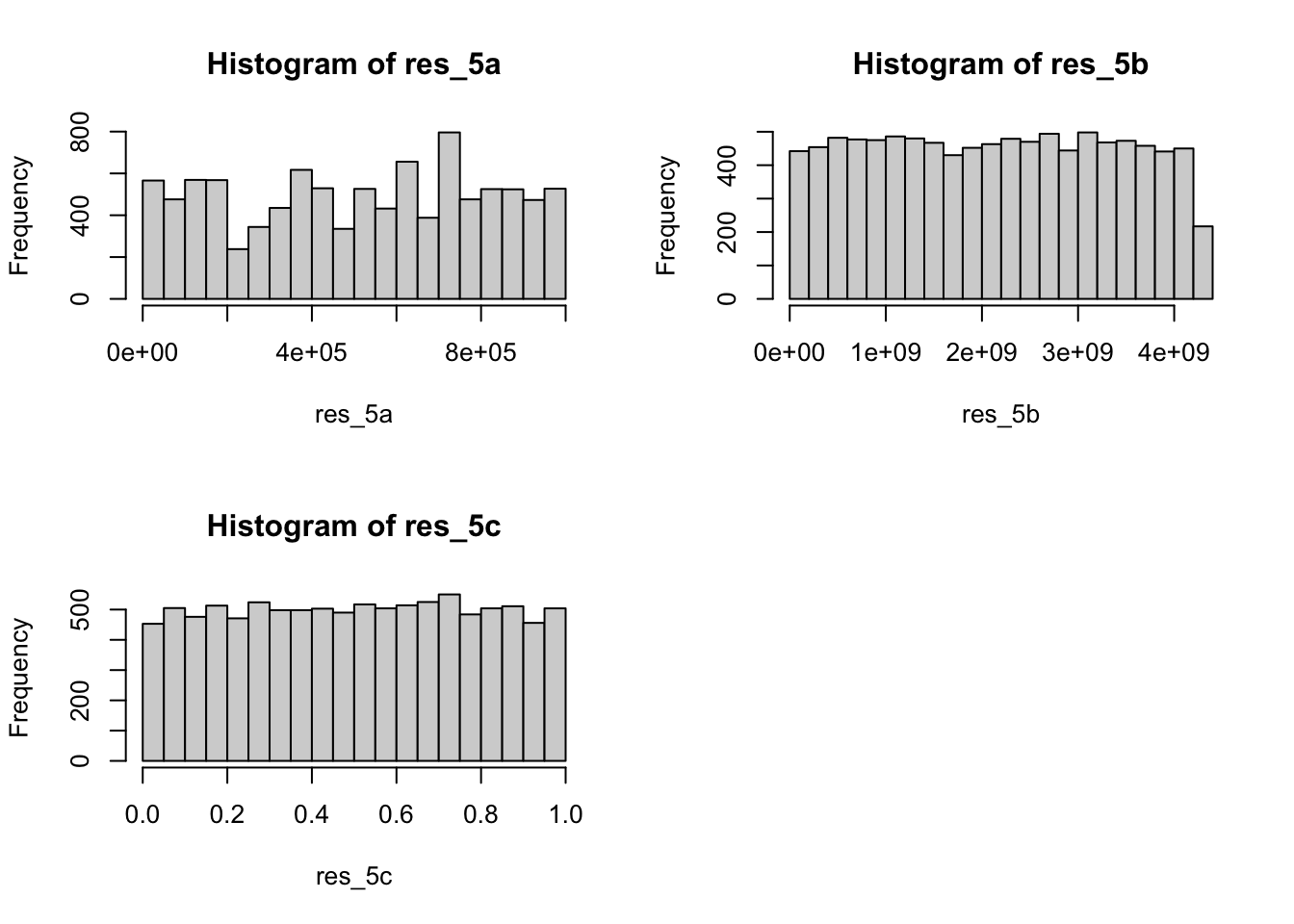# Ex. 6 ## (a) sample and ceiling(runif) • We test a small value (=100) and a large value (=1000000).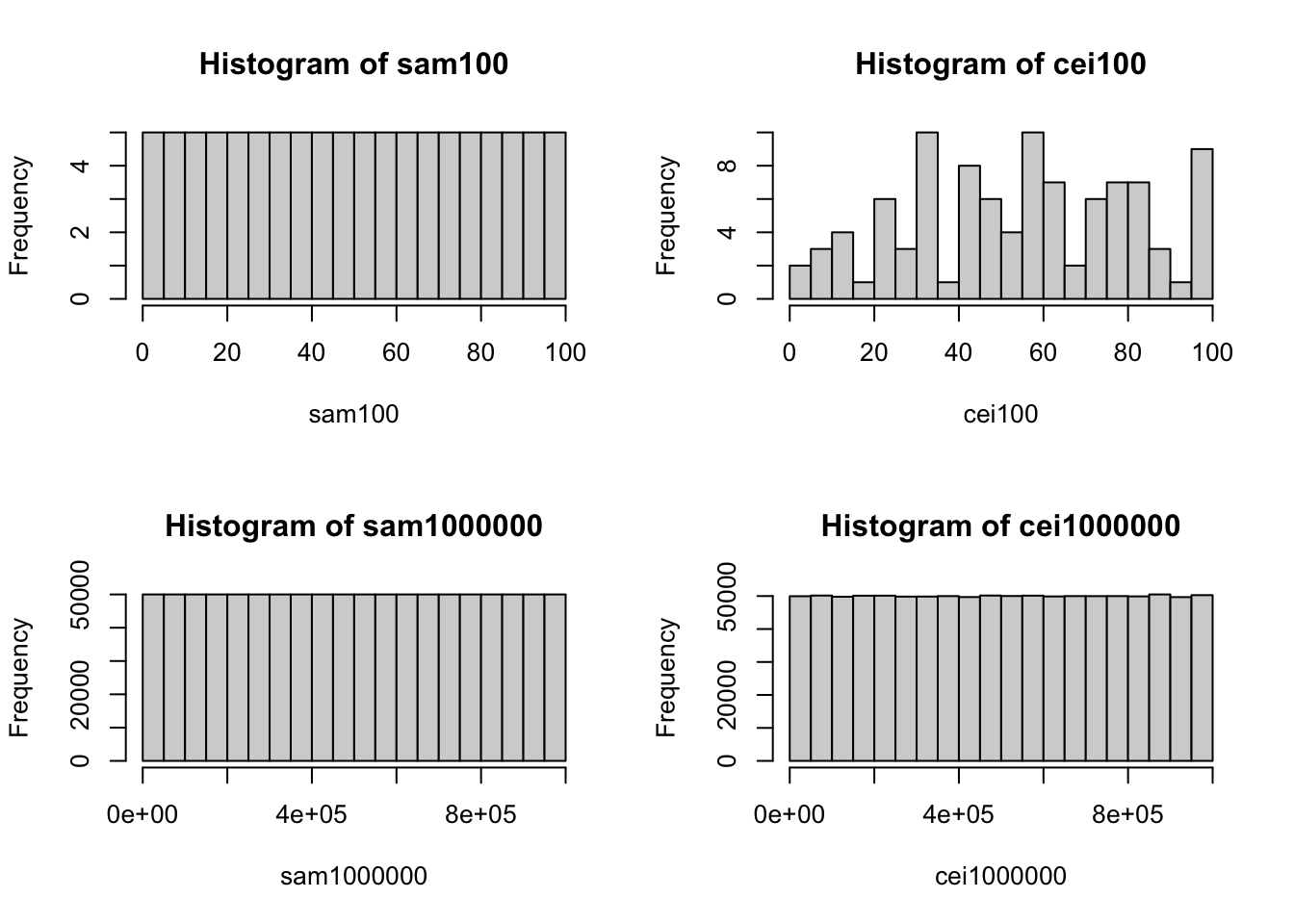• Although these histograms above look very close to uniform distributions. But from the K-S tests, we can find any random numbers created by sample() or ceiling(runif()) are not actually iid from uniform distributions. ## ## One-sample Kolmogorov-Smirnov test ## ## data: sam100 ## D = 1, p-value < 2.2e-16 ## alternative hypothesis: two-sided ## Warning in ks.test(x = cei100, y = "punif"): ties should not be present for the ## Kolmogorov-Smirnov test ## ## One-sample Kolmogorov-Smirnov test ## ## data: cei100 ## D = 1, p-value < 2.2e-16 ## alternative hypothesis: two-sided ## ## One-sample Kolmogorov-Smirnov test ## ## data: sam1000000 ## D = 1, p-value < 2.2e-16 ## alternative hypothesis: two-sided ## Warning in ks.test(x = cei1000000, y = "punif"): ties should not be present for ## the Kolmogorov-Smirnov test ## ## One-sample Kolmogorov-Smirnov test ## ## data: cei1000000 ## D = 1, p-value < 2.2e-16 ## alternative hypothesis: two-sided ## (b) Hand calculators generator • The comment of the gen_6b() function. (1) Create a null vector vec. (2) In the for loop, implement a generators based on uniform and $$\pi$$ (pi+u)^5 %% 1. (3) Save the numbers in the vector vec with the ith place. • Goodness-of-fit test. We generated 10000 random numbers from the gen_6b() function. Check the K-S test D = 0.0090575, p = 0.3848, we can not reject the null hypothesis, i.e. the random numbers do follow the uniform distribution. • Compare to #5. In 5(c) we find only the combination generator can create random numbers followed the uniform distribution. Then we check independence by the runs test (from snpar package). The p-value of both res_5c or res_6b is at the 0.05 significance level, so we can conclude that the numbers form them are random (independent). ## ## One-sample Kolmogorov-Smirnov test ## ## data: res_6b ## D = 0.0090575, p-value = 0.3848 ## alternative hypothesis: two-sided ## ## Approximate runs rest ## ## data: res_5c ## Runs = 5019, p-value = 0.7188 ## alternative hypothesis: two.sided ## ## Approximate runs rest ## ## data: res_6b ## Runs = 4930, p-value = 0.1556 ## alternative hypothesis: two.sided ## (c) $$\phi$$ generator • Replaced $$pi$$ with $$\phi=\dfrac{1+\sqrt{5}}{2}$$. • Check the K-S test D = 0.0066451, p = 0.7692, we can not reject the null hypothesis, i.e. the random numbers do follow the uniform distribution. And from the runs test with p-value = 0.1676, we can find the numbers are random (independent). ## ## One-sample Kolmogorov-Smirnov test ## ## data: res_6c ## D = 0.0066451, p-value = 0.7692 ## alternative hypothesis: two-sided ## ## Approximate runs rest ## ## data: res_6c ## Runs = 4932, p-value = 0.1676 ## alternative hypothesis: two.sided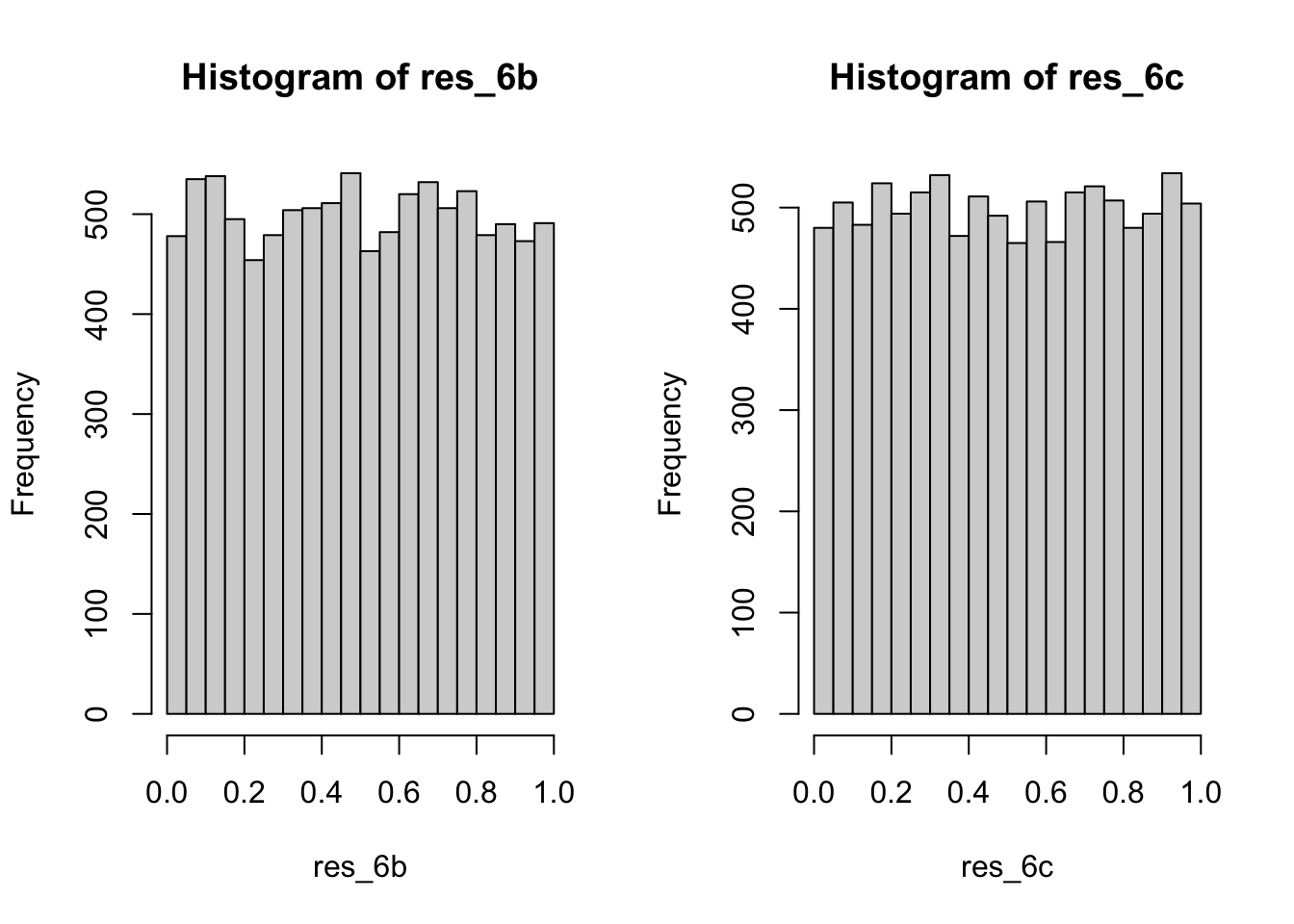# Appendix Note. After R 4.0, some useful new features are built-in. 1. The native pipeline syntax |> replaced the tidy-style pipeline %>%. 2. The shorthand syntax for anonymous functions \(x) is more compact than function(x). ## 1(a) -------- dat1 <- scan('baseball-salaries-2016.txt', what = "character" , sep="\t") dat1a <- matrix(dat1[-c(1:6)], ncol=6, byrow=T) |> data.frame() names(dat1a) <- dat1[1:6] # export data frame write.csv(dat1a, 'dat1a.csv') write.table(dat1a, 'dat1a.txt') ## 1(b) -------- dat1b <- rio::import('baseball-salaries-2016.txt') par(mfrow=c(1,2)) boxplot((W/(W+L)) ~ League, data = dat1b, ylab = 'WPCT' ) boxplot(Salary ($M) ~ League, data = dat1b, ylab = 'Salary')

## 1(c) --------
sala <- dat1b$Salary ($M)
wpct <- dat1b$W/(dat1b$W+dat1b$L) team <- dat1b$Team
cor.test(sala, wpct )

## 1(d) --------
x11()
plot(wpct~sala)
abline( lm(wpct~sala) , lwd=2)
identify(wpct~sala, labels = team)

## 2(a) --------
set.seed(1234)
x_2a = rnorm(20) |> round(2); print(x_2a, width = 65)
length(x_2a) = 15; print(x_2a, width = 65)
length(x_2a) = 18; print(x_2a, width = 65)

## 2(b) --------
set.seed(1234)
x_2a = rnorm(20) |> round(2)
x_2a[x_2a<0] <- NA
print(x_2a, width = 55)

paste0( 'mean: ',mean(x_2a[1:18], na.rm = T) |> round(2) )
paste0( 'median: ', median(x_2a[1:18], na.rm = T) |> round(2) )
paste0( 'var: ', var(x_2a[1:18], na.rm = T) |> round(2) )

## 2(c) --------
x_2a[is.na(x_2a)] <- 0; print(x_2a, width = 55)

## 3(a) 3(b) --------
eq1 <- \(x){ ifelse(x<=3, (x^2-2*x+3), NA) }
eq2 <- \(x){ ifelse(x>3,(x+2*exp(x-3)+1), NA) }

# draw the curves
curve(eq2, from=0, to=6, col='blue', lwd=2,
ylim = c(-5,50),
xlab="x", ylab="f(x)")
curve(eq1, from=0, to=6, col='red', lwd=2,

abline( h=2, lty=2 )
abline( b=(1+2*exp(2)), a=-8*exp(2)+1, lty=2 )

## 4(a) --------
cat(
labels = "Today’s date is: ",
paste( substr(date(),1,10), substr(date(),21,24)),
fill = TRUE
)

cat(
labels = "The time now is: ",
paste( substr(date(),12,19)),
fill = TRUE
)

## 5(a) --------
library('stringr')
# x= initial value, k=length, vec=random number vector
gen_5a <- \(x, k){
vec <- c()
for (i in 1:k){
x <- as.character(x^2) |>
stringr::str_sub(4, 9) |>
as.numeric()
vec[i] <- x
}
return(vec)
}

set.seed(1234)
res_5a <- gen_5a( floor( runif(1)*(10^6) ), 10000 )
print( ks.test(res_5a, y="punif") )

## 5(b)--------
gen_5b <- \(x, k){
vec <- c()
for(i in 1:k){
x <- (69069*x) %% (2^32)
vec[i] <- x
}
return(vec)
}

set.seed(1234)
res_5b <- gen_5b( runif(1), k=10000 )

# ks test
print( ks.test(res_5b, y="punif") )

# chisq test

grp_5b <- \(x, k) {
int <- seq( min(x) , max(x) , (max(res_5b) - min(res_5b))/k )
vec <- c()
for (i in 1:k){
vec[i] <- sum( x<int[i+1] ) - sum( x<int[i] )
}
return(vec)
}

chi_5b <- grp_5b( res_5b, k=10 )
chisq.test( chi_5b, p= rep(1/10, 10) )

## 5(c) --------
gen_5c <- \(x, y, z){
vec <- c()
for(i in 1:10000) {
x <- (171*x) %% 30269
y <- (172*y) %% 30307
z <- (170*z) %% 30323
u <- (x/30269 + y/30307 + z/30323) %% 1
vec[i] <- u
}
return(vec)
}

res_5c <- gen_5c(x=1, y=2, z=3)
print( ks.test(res_5c, y="punif") )

# hist
par( mfrow=c(2,2) )
hist(res_5a, 20); hist(res_5b, 20); hist(res_5c, 20)

## 6(a) --------
set.seed(1234)
# 100
sam100 <- sample(100)
cei100 <- ceiling(runif(100)*100)
sam1000000 <- sample(1000000)
cei1000000 <- ceiling(runif(1000000)*1000000)

# graphical
par(mfrow=c(2,2))
hist(sam100, 20); hist(cei100, 20)
hist(sam1000000, 20); hist(cei1000000, 20)

# test
print(ks.test(x=sam100, y="punif"))
print(ks.test(x=cei100, y="punif"))
print(ks.test(x=sam1000000, y="punif"))
print(ks.test(x=cei1000000, y="punif"))

## 6(b) --------
set.seed(1234)
gen_6b <- \(k){
vec <- c()
u <- runif(1)
for(i in 1:k){
u <- (pi+u)^5 %% 1
vec[i] <- u
}
return(vec)
}

res_6b <- gen_6b(10000)
print( ks.test(res_6b, y="punif") )
snpar::runs.test( res_5c )
snpar::runs.test( res_6b )

# 6(c) --------
set.seed(1234)
gen_6c <- \(k){
vec <- c()
u <- runif(1)
phi <- (1+sqrt(5))/2
for(i in 1:k){
u <- (phi+u)^5 %% 1
vec[i] <- u
}
return(vec)
}

res_6c <- gen_6c(10000)
print( ks.test(res_6c, y="punif") )
snpar::runs.test( res_6c )

# hist
par(mfrow=c(1,2))
hist(res_6b, 20); hist(res_6c, 20)